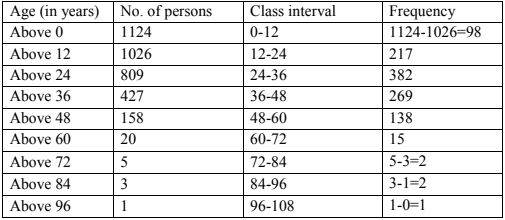# Chapter 22 Tabular Representation of Statistical Data RD Sharma Solutions Exercise 22.2 Class 9 Maths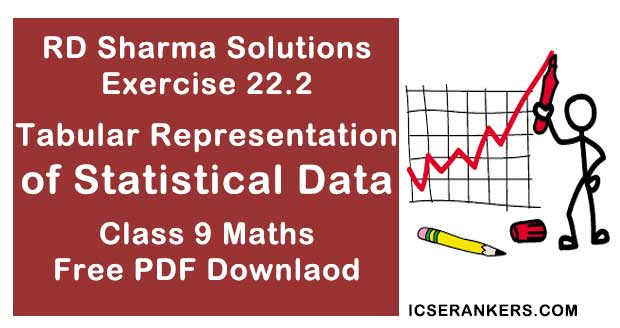Chapter Name RD Sharma Chapter 22 Tabular Representation of Statistical Data Exercise 22.2 Book Name RD Sharma Mathematics for Class 10 Other Exercises Exercise 22.1 Related Study NCERT Solutions for Class 10 Maths

### Exercise 22.2 Solutions

1. Define cumulative frequency distribution.

Solution

Cumulative frequency distribution: A table which displays the manner in which cumulative frequencies are distributed over various classes is called a cumulative frequencies (or) cumulative frequencies distribution table.

2. Explain the difference between a frequency distribution and a cumulative frequency distribution.

Solution

Frequencies table or frequency distribution is a method to represent raw data in the form from which one can easily understand the information contained in a raw data , where as a table which plays the manner in which cumulative frequencies are distributed over various classes is called a cumulative frequency distribution.

3. The marks scored 55 students in a test are given below:

 Marks 0 – 5 5 – 10 10 – 15 15 – 20 20 – 25 25 – 30 30 – 35 No. of students 2 6 13 17 11 4 2
Prepare a cumulative frequency table :
Solution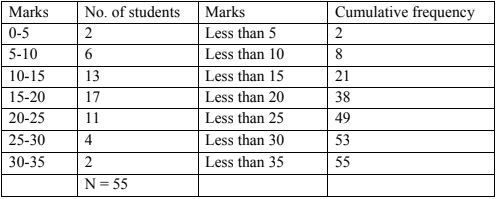4. Following are the ages of  360 patients getting medical treatment in a hospital on a day:
 Age (in years): 10 – 20 20 – 30 30 – 40 40 – 50 50 – 60 60 – 70 No. of Patients : 90 50 60 80 50 30
Construct a cumulative frequency distribution.
Solution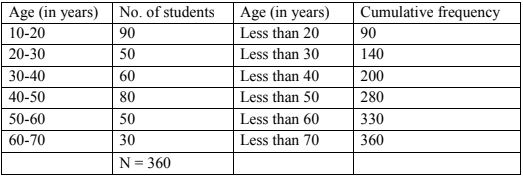5. The water bills (in rupees) of 32 houses in a certain street for the period 1.1.98 to. 31.3.98
are given below:
56, 43, 32, 38, 56, 24, 68, 85, 52, 47, 35, 58, 63, 74, 27, 84, 69, 35, 44, 75, 55, 30, 54, 65, 45,
67, 95, 72, 43, 65, 35, 59.
Tabulate the data and resent the data as a cumulative frequency table using 70-79 as one of
the class intervals.
Solution
The minimum bill is Rs 24.
Maximum bill is Rs 95.
Range = Maximum bill - minimum bill
= 95 - 24 = 71
Given class in terval is 70 - 79, So class size
= 79 - 70 = 9
∴ Number of classes = Range/class size = 71/9 = 7.88
∴ Number of classes = 8
The cumulative frequency distribution table is as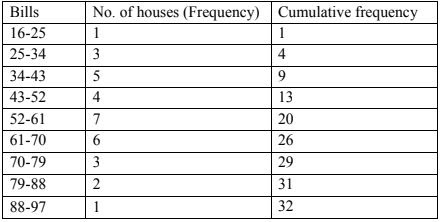6. The number of books in different shelves of a library are as follows:
30, 32, 28, 24, 20, 25, 38, 37, 40, 45, 16, 20
19, 24, 27, 30, 32, 34, 35, 42, 27, 28, 19, 34,
38, 39, 42, 29, 24, 27, 22, 29, 31, 19, 27, 25
28, 23, 24, 32, 34, 18, 27, 25, 37, 31, 24, 23,
43, 32, 28, 31, 24, 23, 26, 36, 32, 29, 28, 21.
Prepare a cumulative frequency distribution table using 45-49 as the last class interval.
Solution
Minimum number of book shelves is 16 and maximum number of book shelves is 45
Range = maximum B.S - Minimum B.S
= 45 - 16 = 29
Given class interval = 45 - 49. So class size
= 49 - 45 = 4
∴ Number of classes = Range/Class size = 29/4 = 7.25
⇒ Number of classes = 8.
The cumulative frequencies distribution is as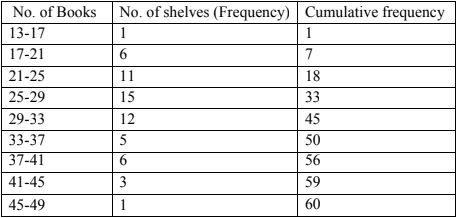7. Given below are the cumulative frequencies showing the weights of 685 students of a school.
Prepare a frequency distribution table.
 Weight (in kg) No. of students Below 25 0 Below 30 24 Below 35 78 Below 40 183 Below 45 294 Below 50 408 Below 55 543 Below 60 621 Below 65 674 Below 70 685
Solution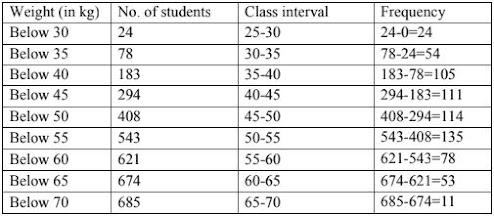8. The following cumulative frequency distribution table shows the daily electricity
consumption (in kW) of 40 factories in an industrial state:
 Consumption(in kW) No. of Factories Below 240 1 Below 270 4 Below 300 8 Below 360 24 Below 390 38 Below 420 40
(i) Represent this as a frequency distribution table.
(ii) Prepare a cumulative frequency table.

Solution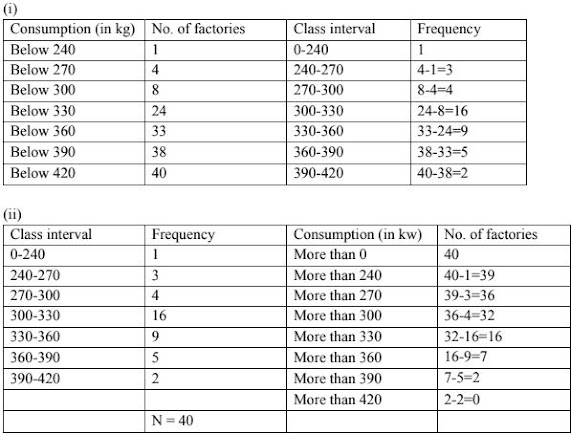9. Given below is a cumulative frequency distribution table showing the ages of people living in a locality:
 Ace in years No. of persons Above 108 0 Above 96 1 Above 84 3 Above 72 5 Above 60 20 Above 48 158 Above 36 427 Above 24 809 Above 12 1026 Above 0 1124
Prepare a frequency distribution table
Solution